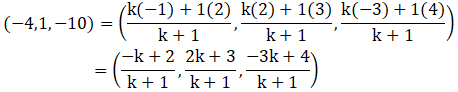Show that the three points A(2, 3, 4), B(-1, 2, -3) and C(-4, 1, -10) are collinear and find the ratio in which C divides AB.

Asked by Sakshi | 1 year ago |  117

##### Solution :-

Given:

The points A (2, 3, 4), B (-1, 2, -3) and C (-4, 1, -10)

Let C divides AB in ratio k: 1

Three points are collinear if the value of k is the same for x, y and z coordinates.

So, m = k and n = 1

A(2, 3, 4), B(-1, 2, -3) and C(-4, 1, -10)

Coordinates of C are:On comparing we get,

$$\dfrac{ [-k + 2] }{[k + 1]}$$ = -4

-k + 2 = -4(k + 1)

-k + 2 = -4k – 4

4k – k = – 2 – 4

3k = -6

k = $$\dfrac{-6}{3}$$

= -2

$$\dfrac{ [2k + 3] }{[k + 1]}$$ = 1

2k + 3 = k + 1

2k – k = 1 – 3

k = – 2

$$\dfrac{ [-3k + 4] }{ [k + 1]}$$ = -10

-3k + 4 = -10(k + 1)

-3k + 4 = -10k – 10

-3k + 10k = -10 – 4

7k = -14

k = $$\dfrac{-14}{7}$$

= -2

The value of k is the same in all three cases.

So, A, B and C are collinear [as k = -2]

We can say that, C divides AB externally in ratio 2:1

Answered by Aaryan | 1 year ago

### Related Questions

#### A(1, 2, 3), B(0, 4, 1), C(-1, -1, -3) are the vertices of a triangle ABC. Find the point in which the bisector of the

A(1, 2, 3), B(0, 4, 1), C(-1, -1, -3) are the vertices of a triangle ABC. Find the point in which the bisector of the angle ∠BAC meets BC.

#### The mid-points of the sides of a triangle ABC are given by (-2, 3, 5), (4, -1, 7) and (6, 5, 3). Find the coordinates

The mid-points of the sides of a triangle ABC are given by (-2, 3, 5), (4, -1, 7) and (6, 5, 3). Find the coordinates of A, B and C.

#### If the points A(3, 2, -4), B(9, 8, -10) and C(5, 4, -6) are collinear, find the ratio in which C divided AB.

If the points A(3, 2, -4), B(9, 8, -10) and C(5, 4, -6) are collinear, find the ratio in which C divided AB.